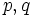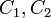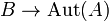# Fusion system-equivalent not implies isomorphic

## Statement

It is possible to have two fusion system-equivalent finite groups$G_1$ and$G_2$ that are not isomorphic.

## Proof

### Idea behind proof

The idea is as follows: the fusion systems induced by the group provide information on the conjugation action on the$p$-subgroups. However, it does not provide clear information regarding which elements of the group are doing the acting. Thus, we need to construct different groups where the actions on each Sylow subgroup are effectively the same but the acting elements are different.

### Generic construction

Consider two primes$p,q$ such that$p$ divides$q - 1$. Let$A$ be the cyclic group of order$q$. Consider a group$B$ of order$p^3$ that has more than one equivalence class up to automorphisms of subgroups of order$p^2$. Let$C_1,C_2$ be representatives of two distinct equivalence classes of such subgroups.

Now define:$G_1 = A \rtimes B$

where the action of$B$ on$A$ is given by a homomorphism$B \to \operatorname{Aut}(A)$ with kernel$C_1$. Note that the image is the unique cyclic subgroup of order$p$ inside$\operatorname{Aut}(A)$, which is cyclic of order$q - 1$.

Also define:$G_2 = A \rtimes B$

where the action of$B$ on$A$ is given by a homomorphism$B \to \operatorname{Aut}(A)$ with kernel$C_2$. Note that the image is the unique cyclic subgroup of order$p$ inside$\operatorname{Aut}(A)$, which is cyclic of order$q - 1$.

We note that:

• Both$G_1$ and$G_2$ have order$p^3q$. Moreover, the$q$-Sylow subgroups of both are isomorphic to$A$ (cyclic of order$q$) and the$p$-Sylow subgroups are isomorphic to$B$ (of order$p^3$).
• The$q$-Sylow subgroups of$G_1$ and$G_2$ are normal in their respective groups, and the fusion system is the same in both cases: In both cases, the action on the subgroup is by the unique subgroup of order$p$ in its automorphism group.
• The fusion systems on the$p$-Sylow subgroups of$G_1$ and$G_2$ are both the inner fusion system: By construction, the$p$-Sylow subgroup is a retract in both cases (i.e., there is a normal p-complement) so this follows.

The upshot is that$G_1$ and$G_2$ are fusion system-equivalent.

On the other hand, they are not isomorphic. The reason is that we can recover$C_i$ up to automorphisms inside$B$ from$G_i$ respectively as follows: first, we recover$A$ as the unique$q$-Sylow subgroup, then we recover$B$ as$G_i/A$ and$C_i = C_G(A)/A$ as a subgroup of$B$.

### Smallest examples of order 24

We take$p = 2, q = 3$ to get examples of order 24. There are two example pairs: